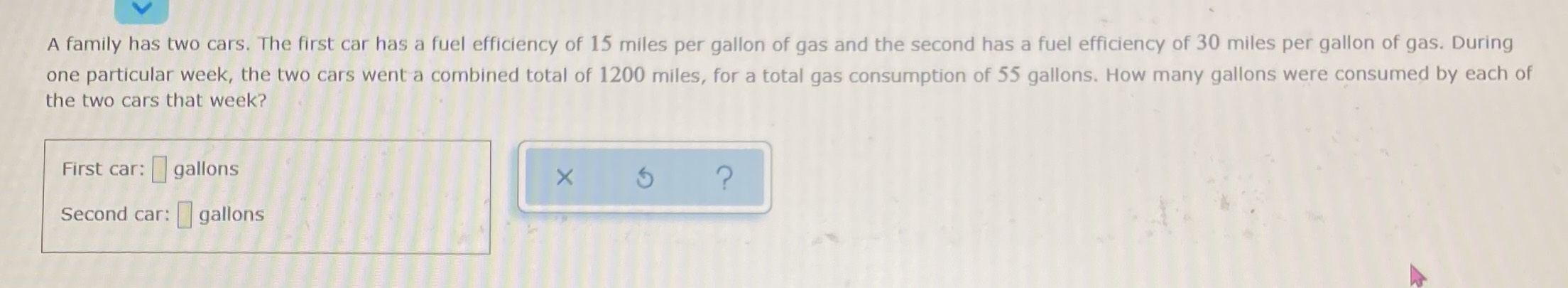### ¿Todavía tienes preguntas de matemáticas?

Pregunte a nuestros tutores expertos
Algebra
PreguntaA family has two cars. The first car has a fuel efficiency of $$15$$ miles per gallon of gas and the second has a fuel efficiency of $$30$$ miles per gallon of gas. During one particular week, the two cars went a combined total of $$1200$$ miles, for a total gas consumption of $$55$$ gallons. How many gallons were consumed by each of the two cars that week? First car: $$\square$$ gallons Second car: $$\square$$ gallons